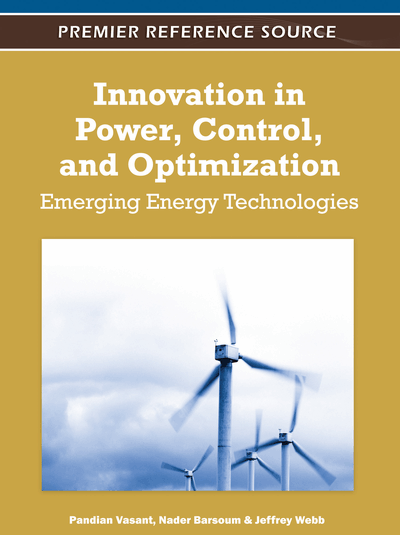# Many-to-Many Assignment Problems: Lagrangian Bounds and Heuristic

Igor Litvinchev (Nuevo Leon State University, Mexico) and Socorro Rangel (São Paulo State University, Brazil)
DOI: 10.4018/978-1-61350-138-2.ch007
Available
\$37.50
No Current Special Offers

## Abstract

Modified Lagrangian bounds and a greedy heuristic are proposed for many-to-many assignment problems taking into account capacity limits for tasks and agents. A feasible solution recovered by the heuristic is used to speed up the subgradient technique to solve the modified Lagrangian dual. A numerical study is presented to compare the quality of the bounds and to demonstrate the efficiency of the overall approach.
Chapter Preview
Top

## Introduction

We examine in this chapter some new Lagrangian heuristics for an important combinatorial optimization problem, a generalized assignment problem. The classical assignment problem involves profit-maximizing assignment of each task to exactly one agent with each agent being assigned to at most one task (a one-to-one assignment). In the generalized assignment problems capacity limits for agents and/or tasks are recognized allowing one-to-many or many-to-many assignment.

To our knowledge, the first generalized assignment problem was studied by De Maio and Roveda (1971). They consider a transportation problem where each demand point must be supplied by exactly one supply point. Here the agents are the supply points and the tasks are the demand points. The requirements of the demand points do not depend on the particular supply point that supplies it, i.e., the requirements are agent-independent. This model was further developed by Srinivasan and Thompson (1972). They made the requirements agent-dependent and are the first ones to propose the model that is known today as the generalized assignment problem. The term generalized assignment problem for this type of problem was first introduced by Ross and Soland (1975).

Cattrysse and Van Wassenhove (1992), Morales and Romeijn (2004) and Pentico (2007) in their survey papers identify a variety of applications in which GAP has been used either directly or as a sub-problem within a broader model type. When the tasks are jobs to be performed, and the agents are computer networks, we obtain the problem described by Balachandran (1976). Another example is the fixed-charge plant location problem, where the agents are capacitated plants and the tasks are customers, where each of the customer demands must be supplied from a single plant (Geoffrion and Graves (1974)). Other applications that have been studied are the location problem (Ross and Soland (1977)), the maximal covering location problem (Klastorin (1979)), various routing problems (Fisher and Jaikumar (1981), Bookbinder and Reece (1988)), assignment in parallel and distributed computing (Pirkul (1986), Bokhari (1987)), R & D planning problems (Zimokha and Rubinshtein (1988)), loading problem in flexible manufacturing systems (Kuhn (1995)), production planning (LeBlanc, Shtub, Anandalingam (1999)).

The real life applications of the assignment models frequently involve large number of tasks and/or agents thus resulting in large-scale optimization problems. However, most large-scale optimization problems exhibit a structure that can be exploited to construct efficient solution techniques. In one of the most general and common forms of a structure the constraints of the problem can be divided into “easy” and “complicated”. In other words, the problem would be an “easy” problem if the complicating constraints could be removed. For example, removing agent constraints in the assignment problems results in independent subproblems corresponding to tasks.

A well-known way to exploit this structure is to form a Lagrangian relaxation with respect to complicating constraints. That is, the complicating constraints are relaxed and a penalty term is added to the objective function to discourage their violation. The optimal value of the Lagrangian problem, considered for fixed multipliers, provides an upper bound (for maximization problem) for the original optimal objective. The problem of finding the best, i.e. bound minimizing Lagrange multipliers, is called the Lagrangian dual. Lagrangian bounds are widely used as a core of many numerical techniques for integer and combinatorial problems, as well as to measure the progress of the main algorithm and derive stopping criteria. In many approximate and heuristic approaches Lagrangian solution is used as a starting or a reference point to construct the algorithm. The literature on Lagrangian relaxation is quite extensive, see, e.g.Lemarechal (2007), Frangioni (2005) and the references therein.

## Complete Chapter List

Search this Book:
Reset